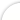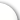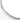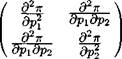Банк рефератов содержит более 364 тысяч рефератов, курсовых и дипломных работ, шпаргалок и докладов по различным дисциплинам: истории, психологии, экономике, менеджменту, философии, праву, экологии. А также изложения, сочинения по литературе, отчеты по практике, топики по английскому.Полнотекстовый поиск.ya-page_js_yes .ya-site-form_inited_no { display: none; }Всего работ: 364139Теги названийРазделыАвиация и космонавтика (304)Административное право (123)Арбитражный процесс (23)Архитектура (113)Астрология (4)Астрономия (4814)Банковское дело (5227)Безопасность жизнедеятельности (2616)Биографии (3423)Биология (4214)Биология и химия (1518)Биржевое дело (68)Ботаника и сельское хоз-во (2836)Бухгалтерский учет и аудит (8269)Валютные отношения (50)Ветеринария (50)Военная кафедра (762)ГДЗ (2)География (5275)Геодезия (30)Геология (1222)Геополитика (43)Государство и право (20403)Гражданское право и процесс (465)Делопроизводство (19)Деньги и кредит (108)ЕГЭ (173)Естествознание (96)Журналистика (899)ЗНО (54)Зоология (34)Издательское дело и полиграфия (476)Инвестиции (106)Иностранный язык (62791)Информатика (3562)Информатика, программирование (6444)Исторические личности (2165)История (21319)История техники (766)Кибернетика (64)Коммуникации и связь (3145)Компьютерные науки (60)Косметология (17)Краеведение и этнография (588)Краткое содержание произведений (1000)Криминалистика (106)Криминология (48)Криптология (3)Кулинария (1167)Культура и искусство (8485)Культурология (537)Литература : зарубежная (2044)Литература и русский язык (11657)Логика (532)Логистика (21)Маркетинг (7985)Математика (3721)Медицина, здоровье (10549)Медицинские науки (88)Международное публичное право (58)Международное частное право (36)Международные отношения (2257)Менеджмент (12491)Металлургия (91)Москвоведение (797)Музыка (1338)Муниципальное право (24)Налоги, налогообложение (214)Наука и техника (1141)Начертательная геометрия (3)Оккультизм и уфология (8)Остальные рефераты (21692)Педагогика (7850)Политология (3801)Право (682)Право, юриспруденция (2881)Предпринимательство (475)Прикладные науки (1)Промышленность, производство (7100)Психология (8692)психология, педагогика (4121)Радиоэлектроника (443)Реклама (952)Религия и мифология (2967)Риторика (23)Сексология (748)Социология (4876)Статистика (95)Страхование (107)Строительные науки (7)Строительство (2004)Схемотехника (15)Таможенная система (663)Теория государства и права (240)Теория организации (39)Теплотехника (25)Технология (624)Товароведение (16)Транспорт (2652)Трудовое право (136)Туризм (90)Уголовное право и процесс (406)Управление (95)Управленческие науки (24)Физика (3462)Физкультура и спорт (4482)Философия (7216)Финансовые науки (4592)Финансы (5386)Фотография (3)Химия (2244)Хозяйственное право (23)Цифровые устройства (29)Экологическое право (35)Экология (4517)Экономика (20644)Экономико-математическое моделирование (666)Экономическая география (119)Экономическая теория (2573)Этика (889)Юриспруденция (288)Языковедение (148)Языкознание, филология (1140)# Реферат: Рrofit function

Название: Рrofit function
Раздел: Рефераты по экономике
Тип: реферат Добавлен 00:21:08 21 января 2009 Похожие работы
Просмотров: 17 Комментариев: 14 Оценило: 2 человек Средний балл: 5 Оценка: неизвестно     Скачать

PROFIT FUNCTION

Given any production set Y, we have seen how to calculate the profit function. 7г(р), which gives us the maximum profit attainable at prices p. The profit function possesses several important properties that follow directly from its definition. These properties are very useful for analyzing profit-maximizing behavior.

Recall that the profit function is, by definition, the maximum profits the firm can make as a function of the vector of prices of the net outputs:

7г(р) = max py у

such that у is in Y.

From the viewpoint of the mathematical results that follow, what is important is that the objective function in this problem is a linear function of prices.

Properties of the profit function

We begin by outlining the properties of the profit function. It is important to recognize that these properties follow solely from the assumption of profit maximization. No assumptions about convexity, monotonicity, or other sorts of regularity are necessary.

Properties of the profit function

1) Nondecreasing in output prices, nonincreasing in input prices. If' p\ > pi for all outputs and p'- < pj for all inputs, then 7r(p') > 7r(p).

2) Homogeneous of degree 1 in p. 7r(£p) £тг(р) for all t > 0.

3) Convex in p. Let p" = fp + (1 - t)p' for 0 < t < 1. Then тг(р") < *7г(р) + (1-*)тг(р').

4) Continuous in p. The function ir(p) is continuous, at least when 7r(p) is well-defined and pt > 0 for i = 1,..., n.

Proof. We emphasize once more that the proofs of these properties follow from the definition of the profit function alone and do not rely on any properties of the technology.

1) Let у be a profit-maximizing net output vector at p, so that 7r(p) = py and let y' be a profit-maximizing net output vector at p' so that 7r(p') = p'y'. Then by definition of profit maximization we have p'y' > p'y. Since p'i > Pi for all i for which j/i > 0 and p\ < pi for all i for which y^ < 0, we also have p'y > py. Putting these two inequalities together, we have " (p') = р'у' > РУ = it(p), as required.

2) Let у be a profit-maximizing net output vector at p, so that py > py' for all y' in Y. It follows that for t > 0, tpy > tpy' for all y' in Y. Hence у also maximizes profits at prices tp. Thus ir(tp) = tpy = tn(p).

3) Let у maximize profits at p, y' maximize profits at p', and y" maximize profits at p". Then we have

тг(р") = p"y" = ( tp + (1 - t ) p ') y " = tpy " + (1 - t ) p ' y ". (3.1)

By the definition of profit maximization, we know that

фу " < Фу = Мр )

(l-t)p'y"<(l-t)p'y' = (l-t)n(p'). Adding these two inequalities and using (3.1). we have

TT(p")<tTr(p) + (l-t)7T(p').

as required.

4) The continuity of 7r(p) follows from the Theorem of the Maximum de scribed in Chapter 27. page 506. I

The facts that the profit function is homogeneous of degree 1 and increasing in output prices are not terribly surprising. The convexity property, on the other hand, does not appear to be especially intuitive. Despite this appearance there is a sound economic rationale for the convexity result, which turns out to have very important consequences.

Consider the graph of profits versus the price of a single output good, with the factor prices held constant, as depicted in Figure 3.1. At the price vector (p*,w*) the profit-maximizing production plan (y*,x*) yields profits p*y* — w*x*. Suppose that p increases, but the firm continues to use the same production plan (y*,x.*). Call the profits yielded by this passive behavior the "passive profit function" and denote it by П(р) = py* — w*x*. This is easily seen to be a straight line. The profits from pursuing an optimal policy must be at least as large as the profits from pursuing the passive policy, so the graph of ir(p) must lie above the graph of H(p). The same argument can be repeated for any price p, so the profit function must lie above its tangent lines at every point. It follows that n(p) must be a convex function.

 PROFITS MP) J U(p) = py- - w'x' MP') ^j^\ S^ P* OUTPUT PRICE

Figure The profit function. As the output price increases, the profit 3.1 function increases at an increasing rate.

The properties of the profit function have several uses. At this point we will satisfy ourselves with the observation that these properties offer several observable implications of profit-maximizing behavior. For example, suppose that we have access to accounting data for some firm and observe that when all prices are scaled up by some factor t > 0 profits do not scale up proportionally. If there were no other apparent changes in the environment, we might suspect that the firm in question is not maximizing profits.

EXAMPLE: The effects of price stabilization

Suppose that a competitive industry faces a randomly fluctuating price for its output. For simplicity we imagine that the price of output will be pi with probability q and pi with probability (1 — q). It has been suggested that it may be desirable to stabilize the price of output at the average price P — 4P\ + (1 ~ 4)P2- How would this affect profits of a typical firm in the industry?

We have to compare average profits when p fluctuates to the profits at the average price. Since the profit function is convex,

Qir(pi) + (1 - 9)t(P2) > f(<7Pi + (1 - q)P2) = 7

Thus average profits with a fluctuating price are at least as large as with a stabilized price.

At first this result seems counterintuitive, but when we remember the economic reason for the convexity of the profit function it becomes clear. Each firm will produce more output when the price is high and less when the price is low. The profit from doing this will exceed the profits from producing a fixed amount of output at the average price.

Supply and demand functions from the profit function

If we are given the net supply function y(p), it is easy to calculate the profit function. We just substitute into the definition of profits to find

t(p) = РУ ( Р )-

Suppose that instead we are given the profit function and are asked to find the net supply functions. How can that be done? It turns out that there is a very simple way to solve this problem: just differentiate the profit function. The proof that this works is the content of the next proposition.

Hotelling's lemma. (The derivative property) Let j/j(p) be the firm's net supply function for good i. Then

Vi\V = —r for г = l,...,n,

OPi

assuming that the derivative exists and that pi > 0.

Proof. Suppose (y*) is a profit-maximizing net output vector at prices (p*). Then define the function

g(p) = тг(р) -ру*.

Clearly, the profit-maximizing production plan at prices p will always be at least as profitable as the production plan y*. However, the plan y* will be a profit-maximizing plan at prices p*, so the function g reaches a minimum value of 0 at p*. The assumptions on prices imply this is an interior minimum.

The first-order conditions for a minimum then imply that

дд(р*) Этг(р*)

—£ = —и Vi = 0 for г = 1,..., п.

dpi дрг

Since this is true for all choices of p*, the proof is done. I

The above proof is just an algebraic version of the relationships depicted in Figure 3.1. Since the graph of the "passive" profit line lies below the graph of the profit function and coincides at one point, the two lines must be tangent at that point. But this implies that the derivative of the profit function at p* must equal the profit-maximizing factor supply at that price: y(p*) = дтт ( р *)/ др .

The argument given for the derivative property is convincing (I hope!) but it may not be enlightening. The following argument may help to see what is going on.

Let us consider the case of a single output and a single input. In this case the first-order condition for a maximum profit takes the simple form

/ Ш _ w = o. (3.2)

ax

The factor demand function x(p, w) must satisfy this first-order condition. The profit function is given by

7r(p, w) = pf(x(p, w)) — Wx(p, w).

Differentiating the profit function with respect to w, say, we have

дтт df(x(p,w)) дх дх

-к- = Р я я w - x ( p , w )

aw ox ow aw

df{x(p,w))

P я w

ox

Ox

Substituting from (3.2), we see that

дтт

- = -x(p,u,)-

The minus sign comes from the fact that we are increasing the price of an input—so profits must decrease.

This argument exhibits the economic rationale behind Hotelling's lemma. When the price of an output increases by a small amount there will be two effects. First, there is a direct effect: because of the price increase the firm will make more profits, even if it continues to produce the same level of output.

But secondly, there will be an indirect effect: the increase in the output price will induce the firm to change its level of output by a small amount. However, the change in profits resulting from any infinitesimal change in output must be zero since we are already at the profit-maximizing production plan. Hence, the impact of the indirect effect is zero, and we are left only with the direct effect.

The envelope theorem

The derivative property of the profit function is a special case of a more general result known as the envelope theorem, described in Chapter 27, page 491. Consider an arbitrary maximization problem where the objective function depends on some parameter a:

M(a) = max f(x,a).

X

The function M(a) gives the maximized value of the objective function as a function of the parameter a. In the case of the profit function a would be some price, x would be some factor demand, and M(a) would be the maximized value of profits as a function of the price.

Let x(a) be the value of x that solves the maximization problem. Then we can also write M(a) = f(x(a), a). This simply says that the optimized value of the function is equal to the function evaluated at the optimizing choice.

It is often of interest to know how M(a) changes as a changes. The envelope theorem tells us the answer:

dM(a) <9/(x, a)

da da =x(a)

This expression says that the derivative of M with respect to a is given by the partial derivative of / with respect to a, holding x fixed at the optimal choice. This is the meaning of the vertical bar to the right of the derivative. The proof of the envelope theorem is a relatively straightforward calculation given in Chapter 27, page 491. (You should try to prove the result yourself before you look at the answer.)

Let's see how the envelope theorem works in the case of a simple one-input, one-output profit maximization problem. The profit maximization problem is

7r(p, w) = max pf(x) wx.

46 PROFIT FUNCTION (Ch. 3)

The a in the envelope theorem is p or w, and M(a) is n(p, w). According to the envelope theorem, the derivative of 7r(p, w) with respect to p is simply the partial derivative of the objective function, evaluated at the optimal choice:

9 ^1 =f(X )\ = /(*(*«,)).

Op x=x(p,w)

This is simply the profit-maximizing supply of the firm at prices (p, w).

Similarly,

dn{p, w)

—я = ~x =-x(p,w),

aw x=x(p,w)

which is the profit-maximizing net supply of the factor.

Comparative statics using the profit function

At the beginning of this chapter we proved that the profit function must satisfy certain properties. We have just seen that the net supply functions are the derivatives of the profit function. It is of interest to see what the properties of the profit function imply about the properties of the net supply functions. Let us examine the properties one by one.

First, the profit function is a monotonic function of the prices. Hence, the partial derivative of 7r(p) with respect to price i will be negative if good i is an input and positive if good i is an output. This is simply the sign convention for net supplies that we have adopted.

Second, the profit function is homogeneous of degree 1 in the prices. We have seen that this implies that the partial derivatives of the profit function must be homogeneous of degree 0. Scaling all prices by a positive factor t won't change the optimal choice of the firm, and therefore profits will scale by the same factor t.

Third, the profit function is a convex function of p. Hence, the matrix of second derivatives of 7r with respect to p—the Hessian matrix—must be a positive semidefinite matrix. But the matrix of second derivatives of the profit function is just the matrix of first derivatives of the net supply functions. In the two-good case, for example, we have(dy, дш \

= dpidp2

I dy2 dy2 I "

\ dp{op2 '

The matrix on the right is just the substitution matrix—how the net supply of good г changes as the price of good j changes. It follows from the properties of the profit function that this must be a symmetric, positive semidefinite matrix.

The fact that the net supply functions are the derivatives of the profit function gives us a handy way to move between properties of the profit function and properties of the net supply functions. Many propositions about profit-maximizing behavior become much easier to derive by using this relationship.

EXAMPLE : The LeChatelier principle

Let us consider the short-run response of a firm's supply behavior as compared to the long-run response. It seems plausible that the firm will respond more to a price change in the long run since, by definition, it has more factors to adjust in the long run than in the short run. This intuitive proposition can be proved rigorously.

For simplicity, we suppose that there is only one output and that the input prices are all fixed. Hence the profit function only depends on the (scalar) price of output. Denote the short-run profit function by ns(p,z) where z is some factor that is fixed in the short run. Let the long-run profit-maximizing demand for this factor be given by z(p) so that the long-run profit function is given by itl(p) = ^s{Pi z {p))- Finally, let p* be some given output price, and let z* = z(p*) be the optimal long-run demand for the 2-factor at p*.

The long-run profits are always at least as large as the short-run profits since the set of factors that can be adjusted in the long run includes the subset of factors that can be adjusted in the short run. It follows that

h(p) = nL (p) - ns (p, 2*) = ns (p, z(p)) - 7TS (p, z*) > 0

for all prices p. At the price p* the difference between the short-run and long-run profits is zero, so that h(p) reaches a minimum at p = p*. Hence, the first derivative must vanish at p*. By Hotelling's lemma, we see that the short-run and the long-run net supplies for each good must be equal at p*.

But we can say more. Since p* is in fact a minimum of h(p), the second derivative of h(p) is nonnegative. This means that

d2 nL (p*) d2 ns (p*,z*) >Q

dp2 dp2 ~

Using Hotelling's lemma once more, it follows that

dVLJP*) dys (p*,z*) = d2 irL {p*) d2 ns (p*,z*) >Q

dp dp dp2 dp2 ~

This expression implies that the long-run supply response to a change in price is at least as large as the short-run supply response at z* = z(p*).

Notes

The properties of the profit function were developed by Hotelling (1932), Hicks (1946), and Samuelson (1947).

Оценить/Добавить комментарий
Имя
Оценка
Комментарии:
Привет студентам) если возникают трудности с любой работой (от реферата и контрольных до диплома), можете обратиться на FAST-REFERAT.RU , я там обычно заказываю, все качественно и в срок) в любом случае попробуйте, за спрос денег не берут)
 Olya 00:10:30 26 августа 2019
.
 . 00:10:29 26 августа 2019
.
 . 00:10:28 26 августа 2019
.
 . 00:10:28 26 августа 2019
.
 . 00:10:27 26 августа 2019

Смотреть все комментарии (14)
Работы, похожие на Реферат: Рrofit function

НазадМенюГлавнаяРефератыБлагодарностиОпросСтанете ли вы заказывать работу за деньги, если не найдете ее в Интернете?

 Да, в любом случае. Да, но только в случае крайней необходимости. Возможно, в зависимости от цены. Нет, напишу его сам. Нет, забью.

Результаты(258741)
Комментарии (3486)Copyright © 2005-2020 BestReferat.ru support@bestreferat.ru реклама на сайте